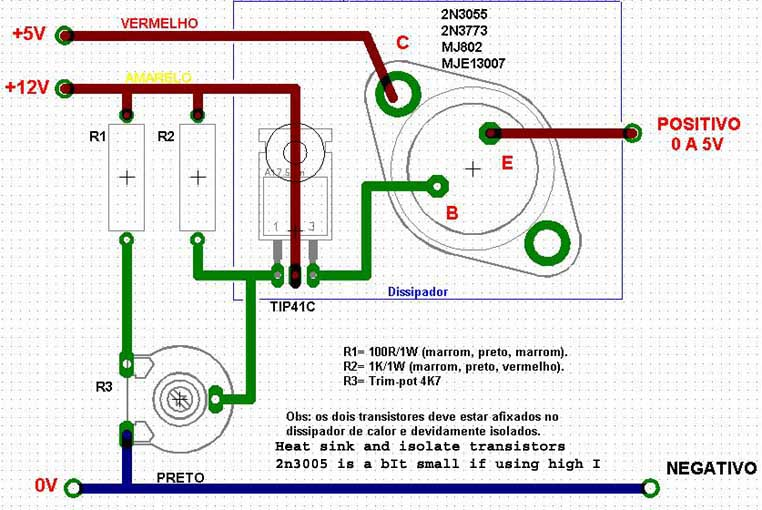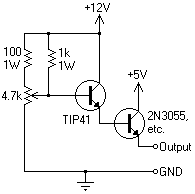# Computer Power supplies

The 5 volt outputs can supply serious current, 25 amps and more. Some are short circuit protected some not, shorting the non protected one's will kill supply. They have a tendency not to come into operation if a load is not connected to the 5 Volt output and sometimes if no load is connected to 12v. Simply connect your cell up first and then turn on the supply or you can put a resistor across the supply to draw about 2 Amps (2.5 Ohms, 10 Watts resistor) or a 12V bulb. This can be removed when you get the cell running. You may need to connect a load (resistor or bulb) permanently to the 12v if you are only using the 5v wires. It varies from unit to unit. Some of the modern units have a 3.3v supply which may suffice if you have a large area of electrodes in your cell.
Sometimes 5 Volts can put too much current into your cell and in that case you can put a power diode in series with the line. The diode will drop about 1 volt giving you 4 volts for the cell. Make sure the Diode is capable of carrying the current and it will also need a heat sink. You can use two (or 3 or 4) diodes if current is still too high. You could also use a high wattage resistor. Remember Ohms law. V = I X R

You can also use the 12 Volt outputs of the supply if the 5 Volts is not driving enough current into you cell. You will have to use diodes or resistors in this case to lower the Voltage seen by the cell or too much current will flow. The 12 Volt outputs cannot put out as much current as the 5 Volt outputs but they are still capable of putting out quite a respectable current. Get a meter to measure the current and don't abuse the supply. It may go into shut down mode if you draw too much current.
A good way to measure the current is to put a low value resistor in series with the line, say 0.01 Ohms. The Voltage measured across this resistor will be related to the current going through it by the formula (Ohms law!!) V = I X R, or if you like I = V/R. If you measure 0.1 Volts across the resistor there will be 10Amps going into cell. Make sure the resistor has high enough Wattage. It should have a minimum wattage = (max. likely current into cell)squared X R. You can make a resistor out of some resistance wire from an old electric fire.

It is possible to put two of theses supplies in parallel and isolate each supply from the rest by putting in a diodes into both leads from the supply's. You may have to use identical units (which may be hard to come by) so that the current is shared equally between units. Do not run the combined units at the max. new possible combined current as the supplies will probably not share the current equally among themselves.
Another alternative for using computer supplies in parallel is to connect all positives to the anode and connect each negative to a seperate Cathode. The Cathodes should be of equal size and spacing from the Anode and from each other. Diodes should also be placed in each supply to help isolate each supply and stop current being driven into one supply from another.
There is lots of info about using computer power supplies as bench power supplies(cell supply) on the net. See here

There is a useful add-on for a computer power supply here.
There is some useful constant current add-on for a computer power supply here (pdf file).

The color code for the wires is:
Red = +5V, Black = Ground (0V), White = -5V, Yellow = +12V, Blue = -12V, Orange = +3.3V, Purple = +5V Standby (not used), Gray = power is on (output), and Green = Turn DC on (input, tie to Black), Brown = 3.3v sense (tie to Orange), +5VSB (ignore).

```Note about computer supplies:

In my setup a modified computer PSU  works perfect. Old UNIX servers
sometimes have PSUs up to 250 A at 5V. While higher output voltage of 6.4 V
instead of 5 V is not really a problem, overriding the over- and
under-voltage protection can be a pain. With PC-PSUs it sometimes helps to
disconnect all other voltages (12V and negatives)at the rectifier diodes to
prevent damage. If there is even a under-voltage protection on these you
should discard the PSU and look for another one. Follow back the massive
copper lines for +5V on the PCB and there will normally be connected one
small line for the loop-back (voltage regulation). Disconnect this line and
reconnect it with a low ohmic voltage divider (i.e 100 Ohm to ground, 28 Ohm
to Output). After that modification the PSU will think to produce 5V while
its 6.4 V. ;-)
```

The following circuit was supplied by Aqua_Fortis_100%
It will allow you to controll the voltage supply using minimum components. It is not a regulator circuit.
Use two 2n3055's in parallel with a small emitter resistor (0.05) in each to balance current if these's transistors are what you have.
This circuit will work better if you leave out R1.Diagram of above.HIT THE BACK BUTTON ON YOUR BROWSER